## Title

Work, energy and power

Work and energy

Whenever a force makes something move, work is done. The amount of work done is equal to the amount of energy transferred. Work, like energy, is measured in joules.When work is done by something, it loses energy; when work is done on something it gains energy.

Gravitational potential energy
A rollercoaster at the top of a slope has stored energy, which is called  gravitational potential energy (GPE), or sometimes potential energy (PE). This is the name used to describe stored energy that an object has because of its position – in this case, higher above the surface of the Earth.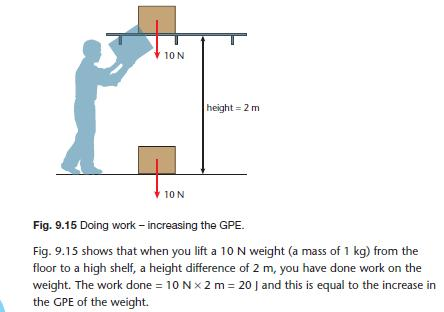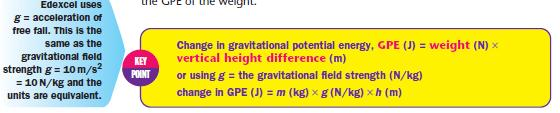On Earth, the gravitational field strength is g = 10 N/kg but on the Moon, because it is smaller g is only about one sixth of this. The size of g increases with the mass of the planet or star. In exams, you will always be given a value to use for g.

Kinetic energy (KE)
An object that is moving has kinetic energy (KE). The energy depends on the mass of the object and on the square of the speed – doubling the speed gives four times the energy.

For example, an air hockey puck, floating on an air table, is almost frictionless. A force does work on the puck by pushing it a small distance. Energy is transferred increasing the kinetic energy of the puck – it speeds up. When the force stops the puck moves at a constant speed across the table – its kinetic energy is now constant.

This explains why stopping distances increase by four times when the speed is doubled – there is four times the kinetic energy to transfer by the braking force.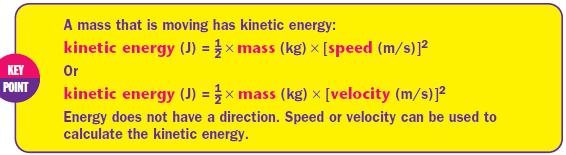Roller coasters and falling

When frictional forces are small enough to be ignored, the transfer of energy between KE and GPE can be used to calculate heights and speeds. Fig. 9.16 shows a car that is driven by a trackside motor to the top of a rollercoaster slope and then freewheels down the slope.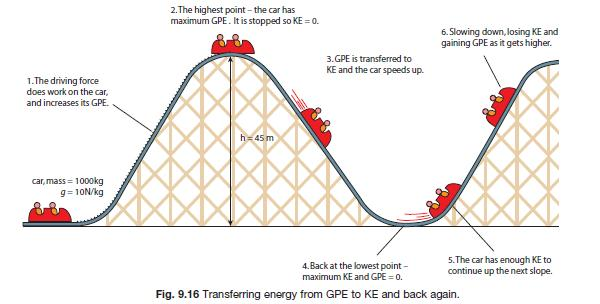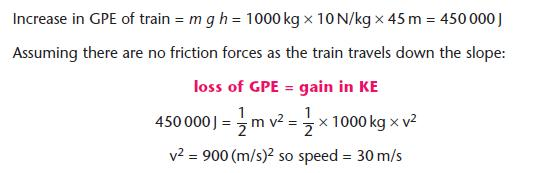REMEMBER THIS EQUATION >>>>

Circular motion
When a rollercoaster travels at a constant speed on a circular part of the track, it is accelerating – its velocity is changing because it is changing direction. There is a resultant force making the train move in a circle. The resultant force and the acceleration are directed towards the centre of the circle. This is always true for any object moving in a circular path.

In Fig. 9.17, a ball on a string moves in a circle. The force in this case is the tension in the string – cut the string and the ball flies off at a tangent – a straight line.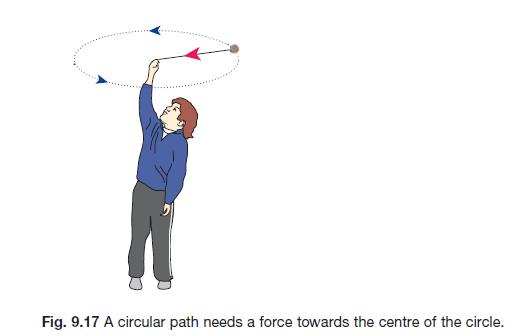Work, friction and conservation of energy
You can only use the relationship ‘gain in KE = loss in GPE’ for a falling object if the drag (air resistance) is small and can be ignored – or if the object is falling in a vacuum. The skydiver in Fig. 9.8  Resistance to motion – friction and drag, eventually reaches terminal velocity. He is still falling, so GPE is being lost, but no KE is being gained. The energy is being used to do work against the frictional force (drag) and the skydiver and surrounding air will heat up.

For an object like the space shuttle, with a lot of KE, heatproof tiles are needed to protect it from the heat resulting from doing work against the drag when it re-enters the Earth’s atmosphere.

Another example is a cyclist travelling at a steady speed. Energy is being transferred by heating the bicycle and surroundings as work is done against friction. No energy is being transferred as KE to the bicycle unless it speeds up.When energy is transferred to the surroundings by heating (for example due to frictional forces) it is no longer useful – but it is not lost. We say it has been dissipated (spread out) as heat. The total energy remains the same. This important result is called the principle of conservation of energy. Energy can be stored and transferred in different ways, but when it is all accounted for, the total amount stays the same.
For AQA, you need to know that some objects can return to their original shape because elastic potential energy is stored in the object when work is done to change its shape.

Power
Power is the rate of energy transfer. This is the work done or energy transferred divided by time.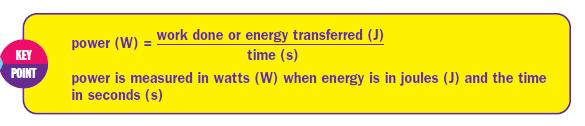Fuel consumption

Fuel consumption is measured in litres per km. (We also uses miles per gallon as a measure of performance – a high figure is good.) Larger vehicles, and those with a greater acceleration have more powerful engines, so that energy can be transferred more quickly from the fuel to do work. The work done = driving force × distance travelled. A more powerful engine can transfer more energy per second – this uses more fuel. Energy from fuel is needed to:

• increase KE
• do work against friction.
When a car brakes, the KE is transferred to heat. Braking and accelerating repeatedly will transfer more energy than travelling at a constant speed. The amount of braking and accelerating will depend on driving style and the road conditions (e.g. single carriageway, lots of bends, traffic lights). At higher speeds, resistive forces are greater and more work is done against friction. If more energy is transferred the fuel consumption is increased (increasing cost, and pollution).